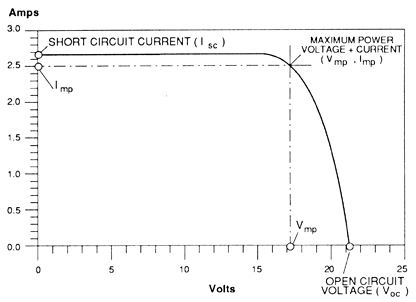A# I-V curve (of a photovoltaic device)A current-voltage (I-V) curve shows the possible combinations of current and voltage output of a photovoltaic (PV) device. A PV device, such as a solar module, produces its maximum current when there is no resistance in the circuit, i.e., when there is a short circuit between its positive and negative terminals. This maximum current is known as the short circuit current and is abbreviated Isc. When the module is shorted, the voltage in the circuit is zero.

Conversely, the maximum voltage occurs when there is a break in the circuit. This is called the open circuit voltage (Voc). Under this condition the resistance is infinitely high and there is no current, since the circuit is incomplete.

These two extremes in load resistance, and the whole range of conditions in between them, are depicted on the I-V curve. Current, expressed in amps, is on the (vertical) y-axis. Voltage, in volts, is on the (horizontal) x-axis .

The power available from a photovoltaic device at any point along the curve is just the product of current and voltage at that point and is expressed in watts. At the short circuit current point, the power output is zero, since the voltage is zero. At the open circuit voltage point, the power output is also zero, but this time it is because the current is zero.

There is a point on the knee of the curve where the maximum power output is located. This maximum power point on our example curve is where the voltage is 17 volts, and the current is 2.5 amps. Therefore the maximum power in watts is 17 volts times 2.5 amps, or 42.5 watts.

The I-V of a PV device curve is based on the device being under standard conditions of sunlight and device temperature. It assumes there is no shading on the device. Standard sunlight conditions on a clear day are assumed to be 1,000 watts of solar energy per square meter (1000 W/m2 or 1 kW/m2). This is sometimes called one sun, or a peak sun. Less than one sun will reduce the current output of the PV device by a proportional amount. For example, if only one-half sun (500 W/m2) is available, the amount of output current is roughly cut in half.